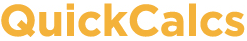1. Select category 2. Choose calculator 3. Enter data 4. View results

## Binomial, Poisson and Gaussian distributions

### Binomial distribution

The binomial distribution applies when there are two possible outcomes. You know the probability of obtaining either outcome (traditionally called "success" and "failure") and want to know the chance of obtaining a certain number of successes in a certain number of trials.

How many trials (or subjects) per experiment?

What is the probability of "success" in each trial or subject?

### Poisson distribution

The Poisson distribution applies when you are counting the number of objects in a certain volume or the number of events in a certain time period. You know the average number of counts, and wish to know the chance of actually observing various numbers of objects or events.

Average number of objects per area (or events per unit time)?

### Gaussian distribution

The Gaussian distribution applies when the outcome is expressed as a number that can have a fractional value. If there are numerous reasons why any particular measurement is different than the mean, the distribution of measurements will tend to follow a Gaussian bell-shaped distribution. If you know the mean and SD of this distribution, you can compute the fraction of the population that is greater (or less) than any particular value.

Mean =   SD =